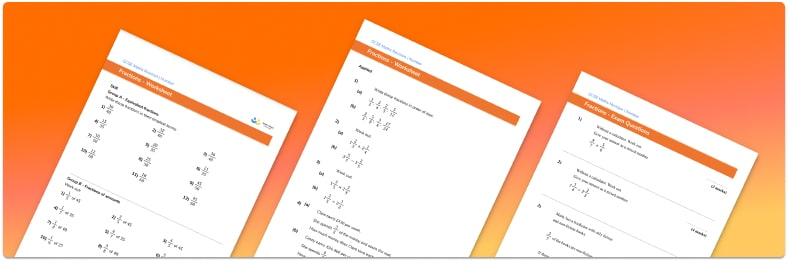# Fraction Worksheet• Section 1 of the printable fraction worksheet contains 36 fraction skills based questions, in 3 groups to support differentiation
• Section 2 contains 4 applied fraction problems with a mix of word problems and deeper problem solving questions
• Section 3 contains 3 foundation and higher level GCSE exam style fraction questions
• Answers and a mark scheme for all fraction questions are provided
• Questions follow variation theory with plenty of opportunities for students to work independently at their own level
• All questions created by fully qualified expert secondary maths teachers
• Suitable for GCSE maths revision for AQA, OCR and Edexcel exam boards

• This field is for validation purposes and should be left unchanged.

You can unsubscribe at any time (each email we send will contain an easy way to unsubscribe). To find out more about how we use your data, see our privacy policy.

### Fractions at a glance

Fractions represent a part of a whole. The denominator of a fraction represents how many equal parts the whole has been divided into and the numerator of the fraction represents how many of those fractional parts we have. For example, the fraction 3 fifths means the whole amount has been divided into 5 equal parts (fifths) and we have 3 of them. Unit fractions have a numerator of 1.

Some fractions are equivalent to each other – they represent the same proportion of a whole. For example, the fractions 1 over 2, 2 over 4, 3 over 6 and 50 over 100  are all equivalent. We may be asked to simplify a fraction and this means finding an equivalent fraction which is made up from smaller numbers (this is sometimes called reducing fractions). Equivalent fractions are useful when converting fractions to percents.

Proper fractions are greater than 0 and less than 1 and can be placed between 0 and 1 on a number line. We also sometimes work with improper fractions, where the numerator is bigger than the denominator, and with mixed numbers (also called mixed fractions) which consist of a whole number and a proper fraction. Improper fractions and mixed numbers are greater than 1 and we can convert between them.

We can find a given fraction of a whole number. To do this, we divide the number by the denominator and then multiply the answer by the numerator.

We can also add, subtract, multiply and divide fractions. When performing fraction addition or subtraction, we need to ensure the fractions have a common denominator. If the fractions have different denominators, we can find equivalent fractions to make the denominators the same. We can then add or subtract the numerators.

When performing fraction multiplication we need to multiply the numerators and multiply the denominators. When dividing fractions we need to turn the second fraction upside down and then multiply them together.

Looking forward, students can then progress to additional number worksheets, for example aor aFor more teaching and learning support on Number our GCSE maths lessons provide step by step support for all GCSE maths concepts.

## Do you have KS4 students who need more focused attention to succeed at GCSE?There will be students in your class who require individual attention to help them succeed in their maths GCSEs. In a class of 30, it’s not always easy to provide.

Help your students feel confident with exam-style questions and the strategies they’ll need to answer them correctly with our dedicated GCSE maths revision programme.

Lessons are selected to provide support where each student needs it most, and specially-trained GCSE maths tutors adapt the pitch and pace of each lesson. This ensures a personalised revision programme that raises grades and boosts confidence.

Find out more